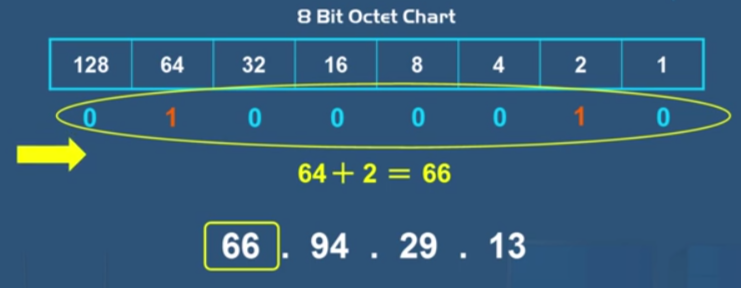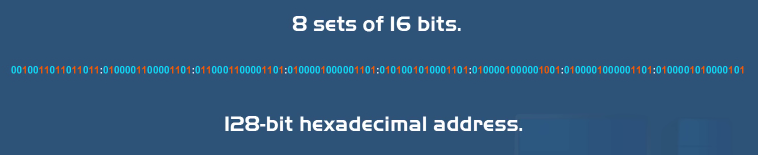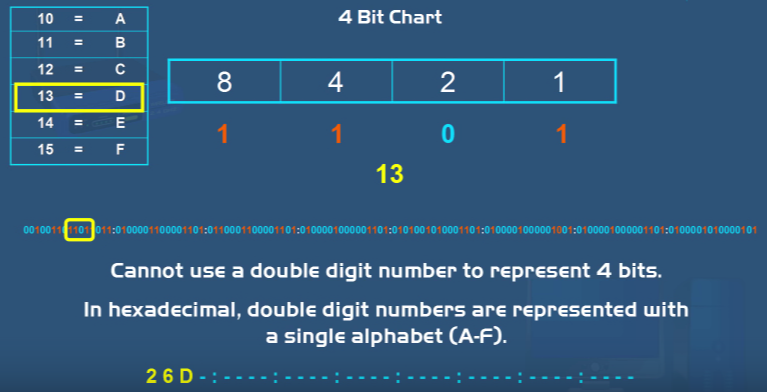Select Page

13

FEBRUARY, 2017

An IP address is a numeric address. It is an identifier for a computer or device on a network. Every device has to have an IP address for communication purposes. The IP address consists of two parts. The first part is the network address and the second part is the host address.

There are also two types of IP addresses the first one is the most common one, it is called IP version 4 (IPv4) and the second type is IP version 6 (IPv6).

### IP version 4 (IPv4)

IPv4 is the current version of IP addresses. It is a 32-bit numeric address written as 4 numbers separated by periods. Each group of numbers that are separated by periods is called an octet. The number range in each octet is 0 – 255. This address version can produce over four billion unique addresses.

In the world of computers and networks the IP address 66.94.29.13 is meaningless. Computers and networks do not read IP addresses in this standard numeric format and that is because they only understand numbers in a binary format.

A binary format is a number that only uses ones and zeros. The binary number the above IP address is 01000010.01011110.00011101.00001101. This binary number is what computers and networking devices actually read.

## How Do We Get The Binary Number From The IP Address?IPv4 is made up of four sets of eight binary bits and these sets are called octets. The bits in each octet are represented by a number. Starting from the left, the first bit has a value of 128 then 64 then 32 and so on, all the way down to one. Each bit on the octet can be either a one or a zero. If the number is a one, then the number that it represents counts if the number is a 0 then the number that it represents does not count. By adding the representatives of the ones and zeros in the octet you can come up with a number ranging from 0 – 255.

For example the first octet in this IP address is 66. To get a binary number out of 66, first you look at the octet chart and you would put ones under the numbers that would add up to the total of 66. You would put a one in the 64 slot and we need two more so we put in a one in the two slot.

Now, all the numbers that we have ones underneath them add up to a total of 66. All of the other bits would be zeros because we don’t need to count them, since we already have our number. The 8 octet number that we got is the binary bit version of 66. The process is the same for the other numbers.

## IP version 6 (IPv6)

When the internet was first developed, programmers did not realize how big it would become. They thought that IPv4 which produces over four billion addresses would be enough. But they were wrong.

IPv6 is the next generation of IP addresses. The main difference between IP version 4 and IPv6is the length of the address. The IP version 4 address is a 32-bit numeric address whereas IPv6is a 128-bit hexadecimal address.

Hexadecimal uses both numbers and alphabets in the address. With this type of address, IPv6can produce an unbelievable 340 undeCillian IP addresses. That is the number 340 with thirty six digits after it. As you might have guessed, IPv6is more than enough for the foreseeable future.As stated before IP version this is a 128-bit hexadecimal address. It is made up of eight sets of 16 bits with the eight sets separated by colons.

In an IPv6IP address each hexadecimal character represents 4 bits, so we have to convert 4 bits at a time to get one hexadecimal character. Starting from the beginning we convert the first four bits and put those bits against that a 4 bit chart which includes an 8, 4, 2 and a 1.If we count the numbers that have ones underneath them you wind up with a 2. So 2 is the first hexadecimal character in this IPv6 address. For the next four binary numbers we get a 6 using the same process. So a 6 is the second hexadecimal character in this IP address.For the next set of four bits, and if we add all the numbers that we have ones underneath them we get a total of 13. But the problem is since 13 is a double digit number we cannot use a double digit number to represent four bits. This is because in a hexadecimal format double-digit numbers have to be represented with a single alphabet which is A through F.

In this case we have to use another chart for any four bits that the sum is 10 or higher. If the sum was 10 then we would use the letter A or if the sum was 11 then we would use a B and so on. In this case our Sum is 13 so for the third character in our binary number we would put a D.For our last four set of bits and if we add the ones we get 11. So we have a double-digit character again which means that we have to convert it to a single character alphabet. If we look at our chart, 11 converts to a B. So the first 16 bits of this binary IPv6 address converts to the hexadecimal address as 26DB.